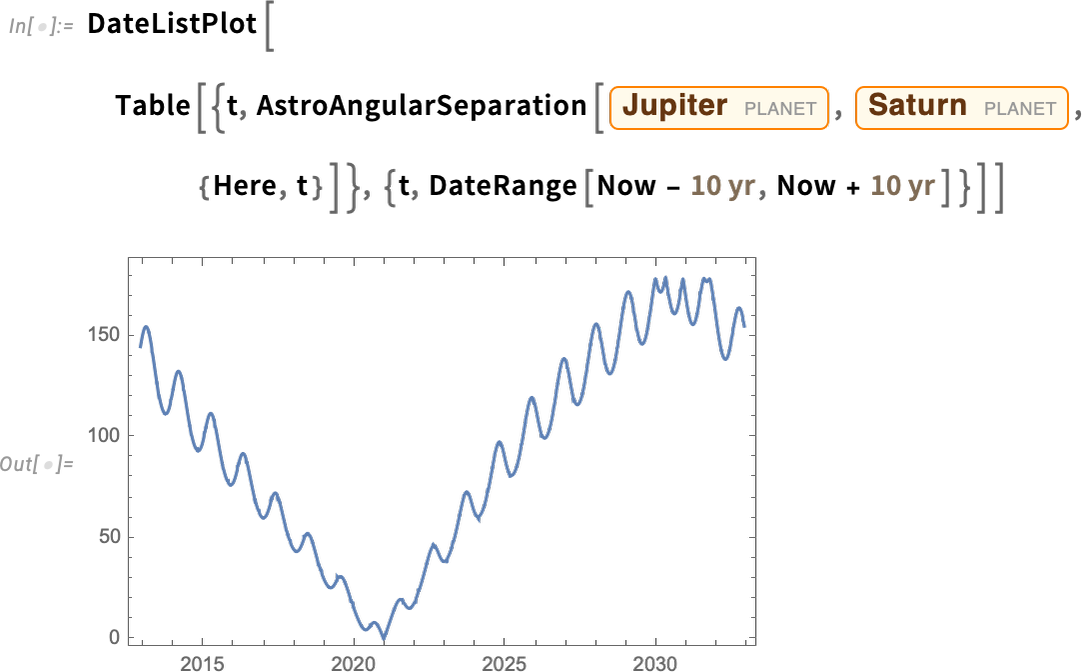Browse by Topic
Related Topics

# New in 13.2: Introducing Astro Computation

Last year we released Version 13.1 of the Wolfram Language. Here are the updates in astro computation since then, including the latest features in 13.2.

### Introducing Astro Computation

Astronomy has been a driving force for computation for more than 2000 years (from the Antikythera device on)… and in Version 13.2 it’s coming to Wolfram Language in a big way. Yes, the Wolfram Language (and Wolfram|Alpha) have had astronomical data for well over a decade. But what’s new now is astronomical computation fully integrated into the system. In many ways, our astro computation capabilities are modeled on our geo computation ones. But astro is substantially more complicated. Mountains don’t move (at least perceptibly), but planets certainly do. Relativity also isn’t important in geography, but it is in astronomy. And on the Earth, latitude and longitude are good standard ways to describe where things are. But in astronomy—especially with everything moving—describing where things are is much more complicated. Oh, and there’s the question of where things “are,” versus where things appear to be—because of effects ranging from light-propagation delays to refraction in the Earth’s atmosphere.

The key function for representing where astronomical things are is AstroPosition. Here’s where Mars is now: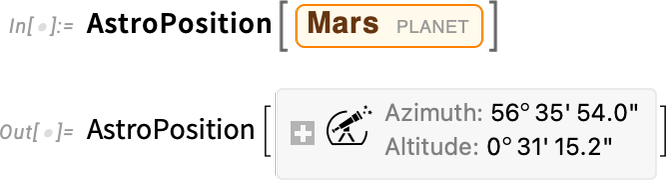What does that output mean? It’s very “here and now” oriented. By default, it’s telling me the azimuth (angle from north) and altitude (angle above the horizon) for Mars from where Here says I am, at the time specified by Now. How can I get a less “personal” representation of “where Mars is”? Because if even I just reevaluate my previous input now, I’ll get a slightly different answer, just because of the rotation of the Earth: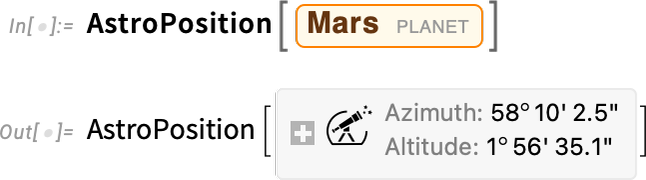One thing to do is to use equatorial coordinates, that are based on a frame centered at the center of the Earth but not rotating with the Earth. (One direction is defined by the rotation axis of the Earth, the other by where the Sun is at the time of the spring equinox.) The result is the “astronomer-friendly” right ascension/declination position of Mars: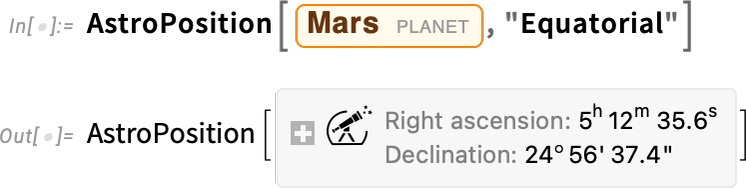And maybe that’s good enough for a terrestrial astronomer. But what if you want to specify the position of Mars in a way that doesn’t refer to the Earth? Then you can use the now-standard ICRS frame, which is centered at the center of mass of the Solar System: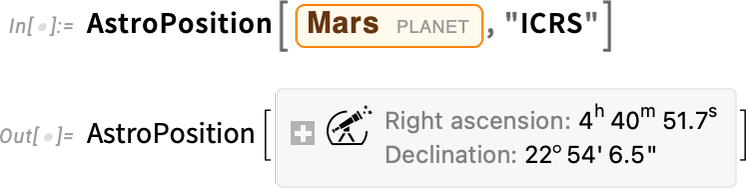Often in astronomy the question is basically “which direction should I point my telescope in?”, and that’s something one wants to specify in spherical coordinates. But particularly if one’s “out and about in the Solar System” (say thinking about a spacecraft), it’s more useful to be able to give actual Cartesian coordinates for where one is: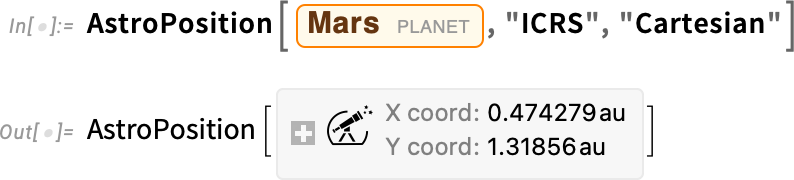And here are the raw coordinates (by default in astronomical units):AstroPosition is backed by lots of computation, and in particular by ephemeris data that covers all planets and their moons, together with other substantial bodies in the Solar System: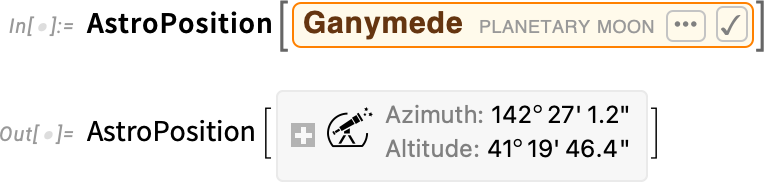By the way, particularly the first time you ask for the position of an obscure object, there may be some delay while the necessary ephemeris gets downloaded. The main ephemerides we use give data for the period 2000–2050. But we also have access to other ephemerides that cover much longer periods. So, for example, we can tell where Ganymede was when Galileo first observed it: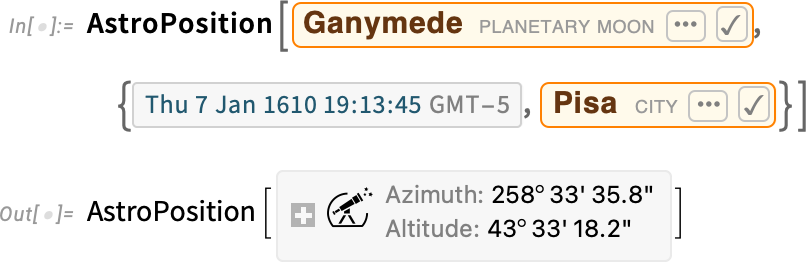We also have position data for more than 100,000 stars, galaxies, pulsars and other objects—with many more coming soon: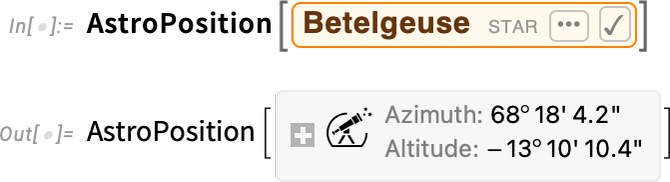Things get complicated very quickly. Here’s the position of Venus seen from Mars, using a frame centered at the center of Mars: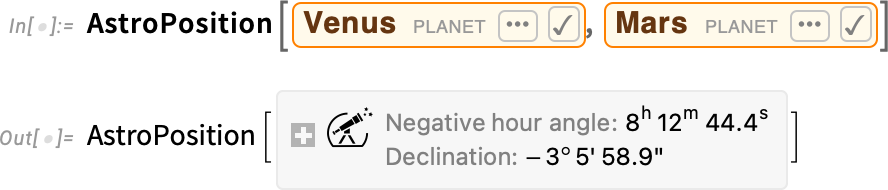If we pick a particular point on Mars, then we can get the result in azimuth-altitude coordinates relative to the Martian horizon:Another complication is that if you’re looking at something from the surface of the Earth, you’re looking through the atmosphere, and the atmosphere refracts light, making the position of the object look different. By default, AstroPosition takes account of this when you use coordinates based on the horizon. But you can switch it off, and then the results will be different—and, for example, for the Sun at sunset, substantially different: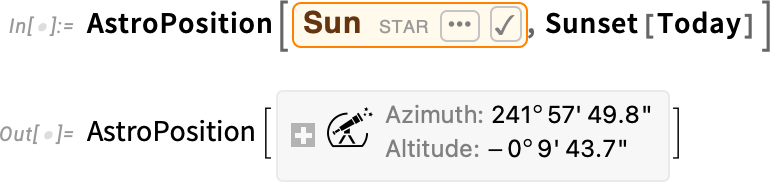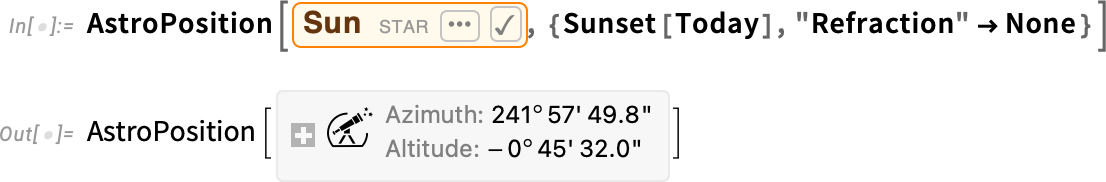And then there’s the speed of light, and relativity, to think about. Let’s say we want to know where Neptune “is” now. Well, do we mean where Neptune “actually is”, or do we mean “where we observe Neptune to be” based on light from Neptune coming to us? For frames referring to observations from Earth, we’re normally concerned with the case where we include the “light time” effect—and, yes, it does make a difference: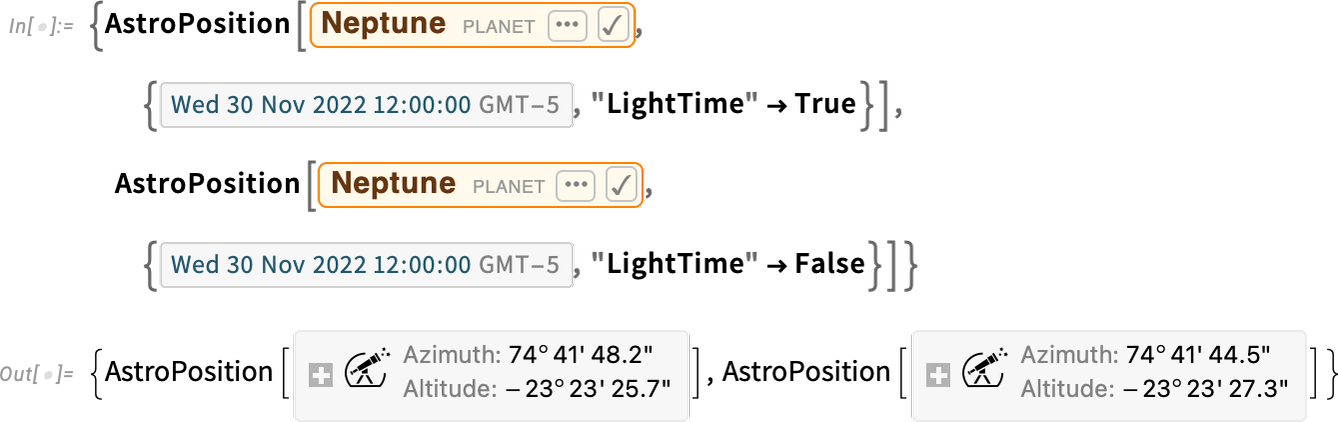OK, so AstroPosition—which is the analog of GeoPosition—gives us a way to represent where things are, astronomically. The next important function to discuss is AstroDistance—the analog of GeoDistance.

This gives the current distance between Venus and Mars:This is the current distance from where we are (according to Here) and the position of the Viking 2 lander on Mars:This is the distance from Here to the star τ Ceti:To be more precise, AstroDistance really tells us the distance from a certain object, to an observer, at a certain local time for the observer (and, yes, the fact that it’s local time matters because of light delays):And, yes, things are quite precise. Here’s the distance to the Apollo 11 landing site on the Moon, computed 5 times with a 1-second pause in between, and shown to 10-digit precision: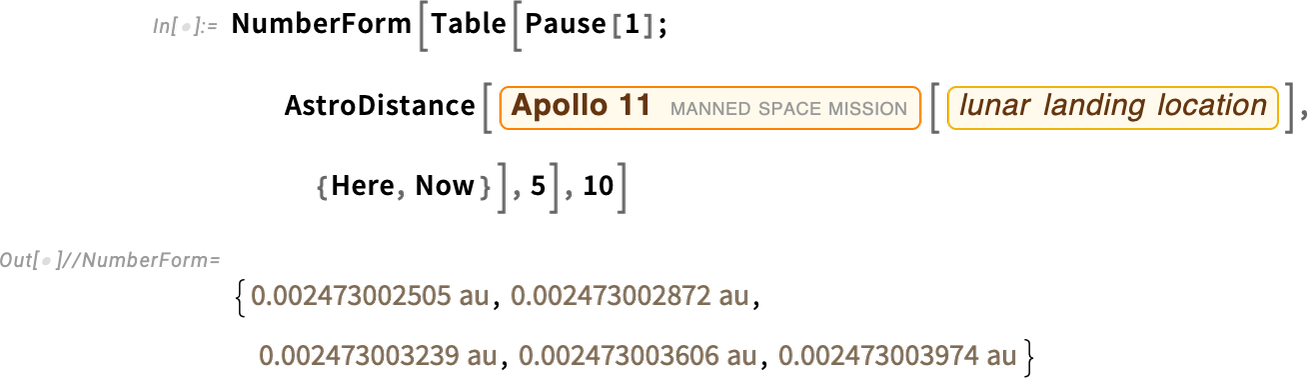This plots the distance to Mars for every day in the next 10 years: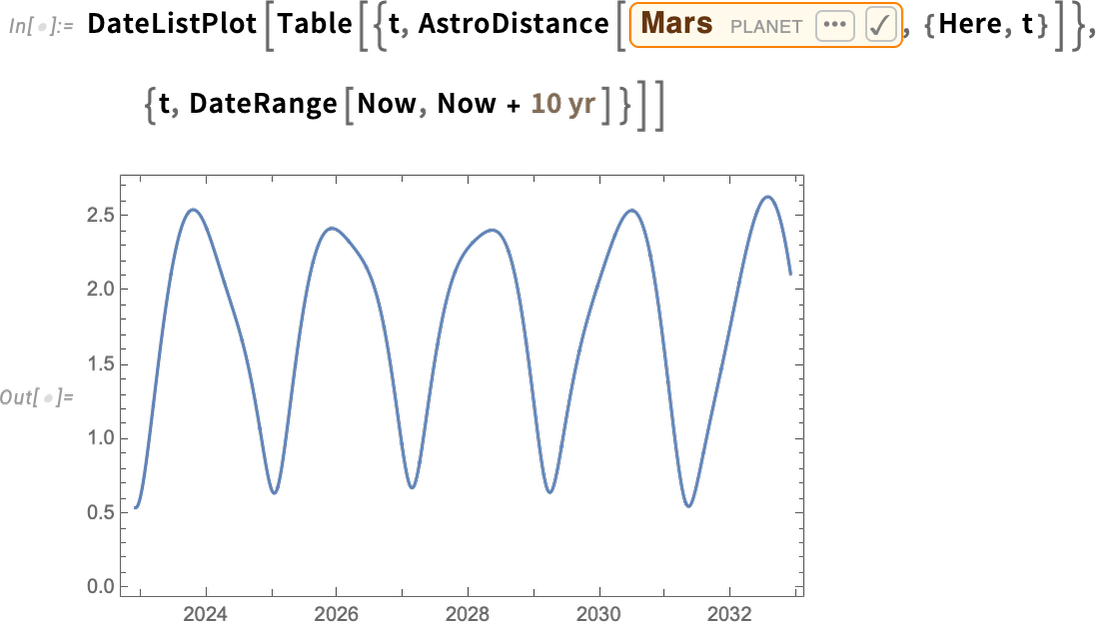Another function is AstroAngularSeparation, which gives the angular separation between two objects as seen from a given position. Here’s the result from Jupiter and Saturn (seen from the Earth) over a 20-year span: